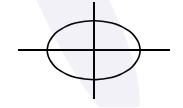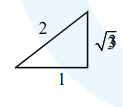Deepak Scored 45->99%ile with Bounce Back Crack Course. You can do it too!

# If the normal to the ellipse

Question:

If the normal to the ellipse $3 x^{2}+4 y^{2}=12$ at a point $P$ on it is parallel to the line, $2 x+y=4$ and the tangent to the ellipse at $P$ passes through $Q(4,4)$ then $P Q$ is equal to :

1. $\frac{\sqrt{221}}{2}$

2. $\frac{\sqrt{157}}{2}$

3. $\frac{\sqrt{61}}{2}$

4. $\frac{5 \sqrt{5}}{2}$

Correct Option: , 4

Solution:$3 x^{2}+4 y^{2}=12$

$x=2 \cos \theta, y=\sqrt{3} \sin \theta$

Let $\mathrm{P}(2 \cos \theta, \sqrt{3 \sin \theta})$

Equation of normal is $\frac{\mathrm{a}^{2} \mathrm{x}}{\mathrm{x}_{1}}-\frac{\mathrm{b}^{2} \mathrm{y}}{\mathrm{y}_{1}}=\mathrm{a}^{2}-\mathrm{b}^{2}$

$2 x \sin \theta-\sqrt{3} \cos \theta y=\sin \theta \cos \theta$

Slope $\frac{2}{\sqrt{3}} \tan \theta=-2 \quad \therefore \tan \theta=-\sqrt{3}$

Equation of tangent is

it passes through $(4,4)$$3 x \cos \theta+2 \sqrt{3} \sin \theta y=6$

$12 \cos \theta+8 \sqrt{3} \sin \theta=6$

$\cos \theta=-\frac{1}{2}, \sin \theta=\frac{\sqrt{3}}{2} \therefore \theta=120^{\circ}$

Hence point $\mathrm{P}$ is $\left(2 \cos 120^{\circ}, \sqrt{3} \sin 120^{\circ}\right)$

$\mathrm{P}\left(-1, \frac{3}{2}\right), \mathrm{Q}(4,4)$

$\mathrm{PQ}=\frac{5 \sqrt{5}}{2}$